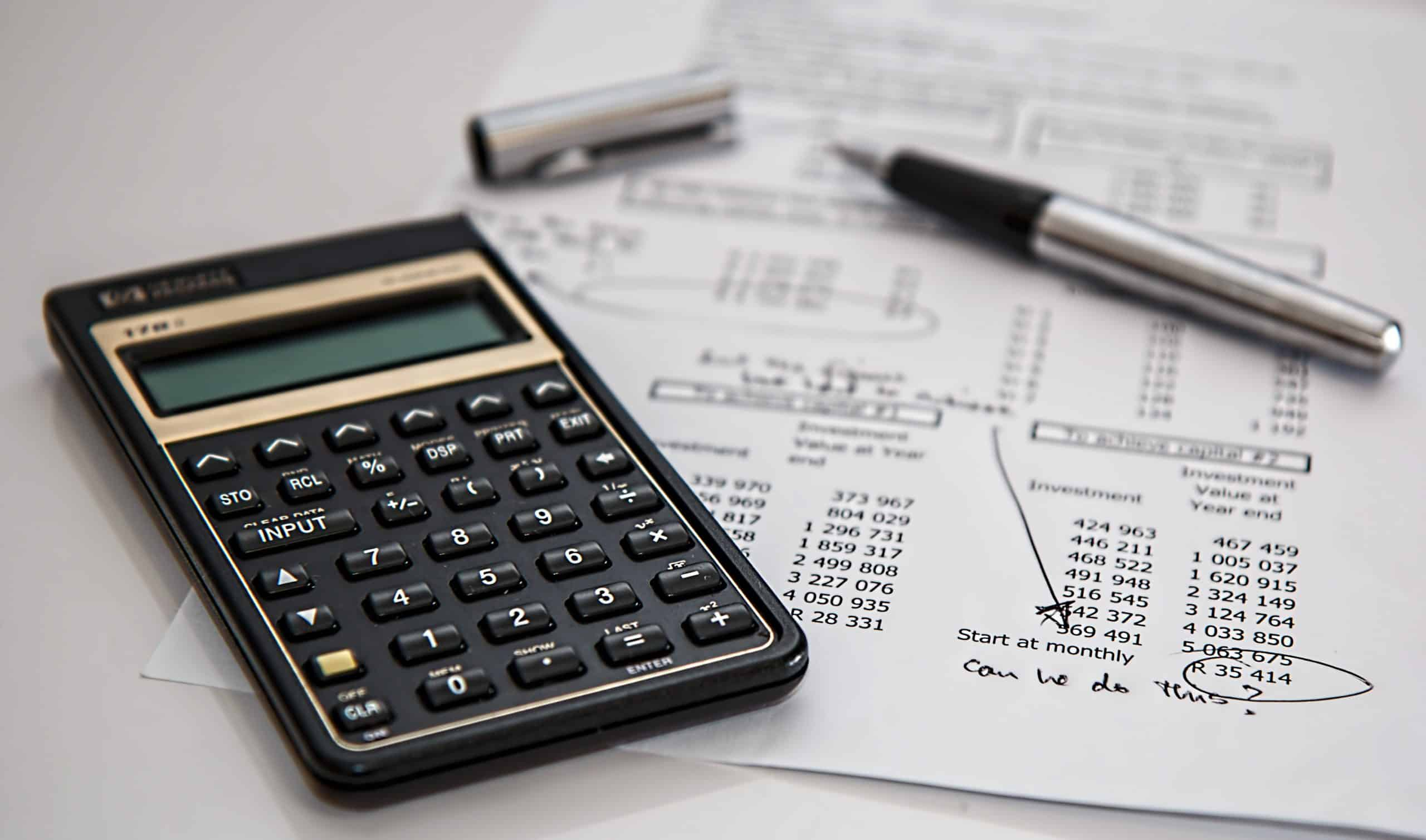# Book Value Per Share Formula

#### BySteve Burns

Jun 12, 2020Book value is a fundamental metric used to measure the intrinsic value of a company with the ratio of price/book value. This valuation metric can at times create a price support level for stock prices as this is the valuation that value investors will come in as buyers.

To quantify the current value of a publicly traded company all assets are added and then subtracted from total liabilities (Total assets – total liabilities) to calculate a approximate total fundamental value of the company.

This ‘book value’ is then divided by the total number of outstanding shares of the company of the share float including all publicly traded and private held ownership. The market price value calculated based on the net value of the enterprise is divided by the total share float is the book value per share.

The book value per share is calculated by dividing a company’s total equity value by its total number of shares outstanding.

If the total value of a company is \$10 million, and it has 1 million shares of stock outstanding: \$10 million/1 million: it has a \$10 per share book value. Now, the shares will trade at whatever value the market gives them based on future expectations being good or bad and technical price action patterns, the book value is only the current fundamental intrinsic value of the company based on assets.

The book value can reference a business’ true total equity worth, it is also used as a ‘per share value’ when the corporate balance sheet equity value is then divided by the amount of outstanding shares at any given time.

In the case of a bankruptcy and liquidation, the book value can be extracted by selling a company’s assets and the money that is left after all of the company debts are paid is the extracted value.

Buying a company with a low valuation like a a price to book value that equals one, is a great risk/reward ratio as the company can theoretically be liquidated for the buying price.

Stock shares in low margin, high labor industrial industries tend to sell at lower price/book ratios as they create lower earnings for each dollar of assets. Businesses using technology, digital assets, websites, or monetizing human capital can cash flow higher earnings per dollar of assets, and most often trade at higher price/book ratios.

A ‘diluted per share value’ can happen when the total equity in the company is increased by the exercise of stock options created and given to executives, new issue of preferred shares, warrants, or secondary offerings. When the adding of additional stock to the share float the total book value has to  it is divided by the number of shares that has been increased by those added.

Things that change the book value of a company:

1. The sale of assets of a business unit.
2. Share buybacks that reduce the amount of outstanding shares increases value of the remaining shares by lowering the multiple of shares divided by the company value.
3. Paying dividends reduces cash on hand.
4. Drop in earnings power reduces book value as discounted future cash flows drop.
5. Dilution of outstanding shares through issuing more shares.

Book value = assets – liabilities.

Book value per share = book value / outstanding shares.

Price to book = share price / book value per share.